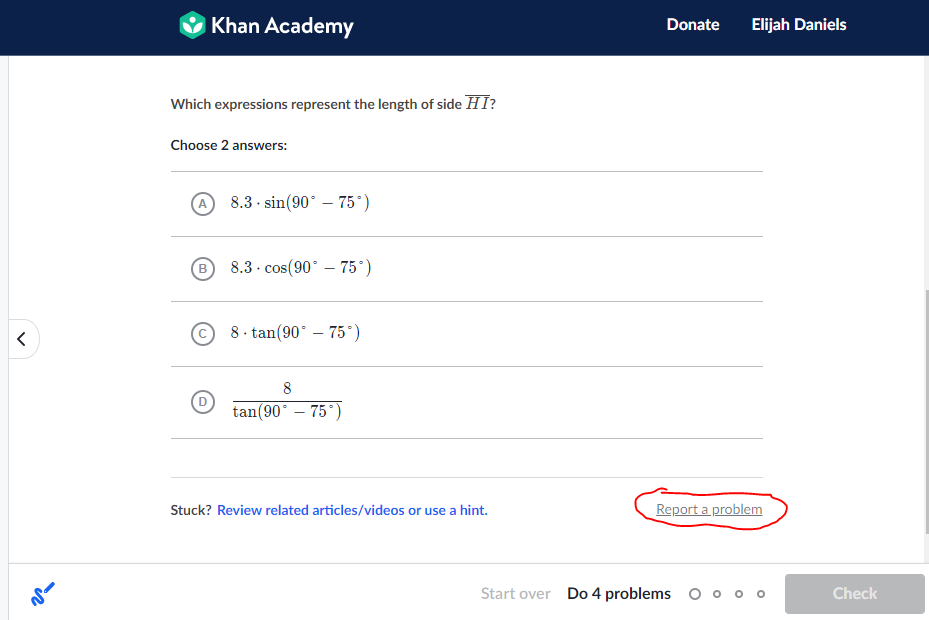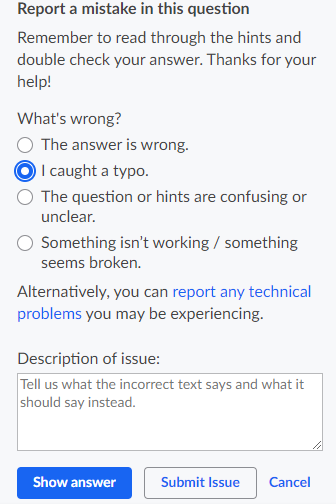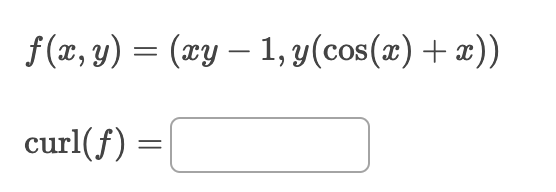How can we help?

• Hello,

I'm sorry this is happening! I am also in the process of working through the multivariable calculus course and definitely understand this kind of frustration. Recently, I also found what I thought to be a typo with the answer choices (the function had 3 variables, but the answer only ordered pairs of 2 variables). When this sort of issue arises, the best course of action is to report it from the quiz itself.On the quiz, click "Report a problem" in the lower right, above the check box.From here, you can select which ever choice best describes the issue at hand, and leave a description of what should be corrected. This way the issue can be sent directly to the team for inspection.

Thank you for the feedback! Hope this helps!

~ Elijah

• I searched for the problem you reported and I think you are referring to this one?In this problem, the entire cos(x) + x is being multiplied by y, so if you first distribute then you would get ycos(x) + xy.

The partial derivative with respect to x is then -ysin(y) + y as correctly stated in the problem hints.

I think you were taking the partial derivative of ycos(x) + x, which is not correct, since that does not properly distribute the factor across both terms in the expression.

Does that make sense? Let me know if there's anything else I can do to help!